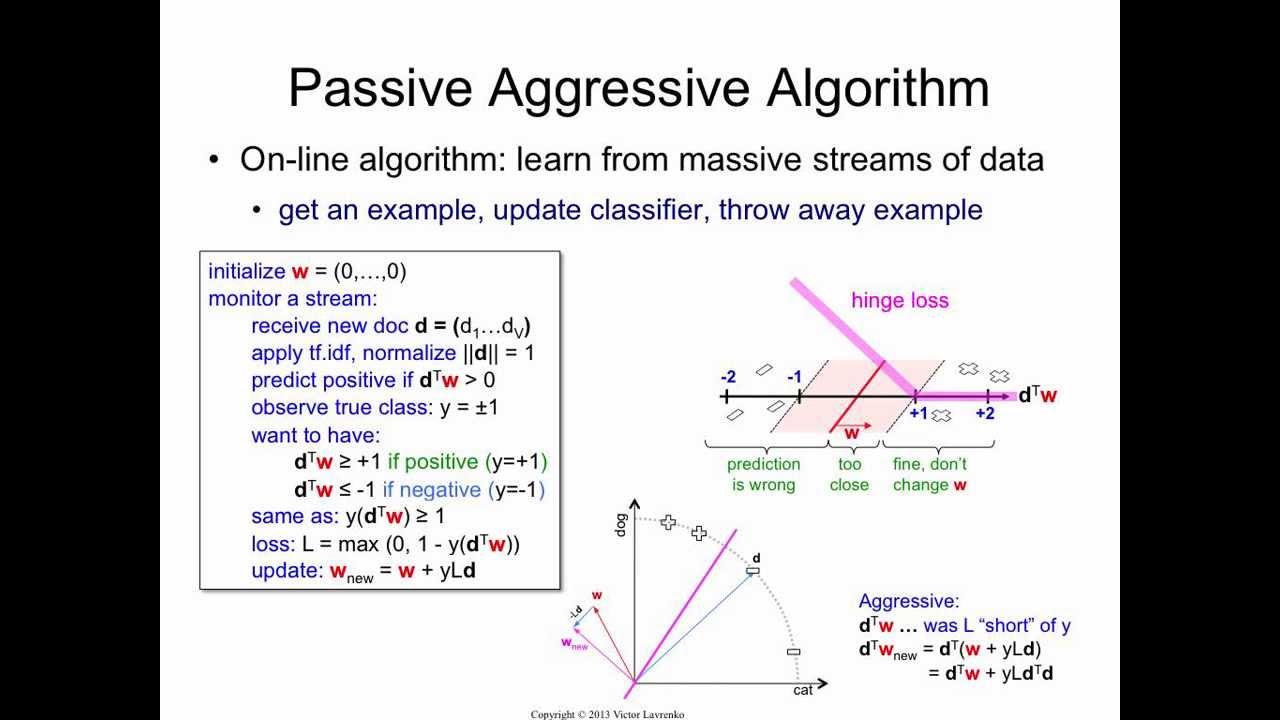# Draw a function with margin area in Latex

Is there an easy way to draw something like the image on the right side in Latex? The one that says "hinge loss" but the hinge loss function shouldn't appear. Or is it too complex for drawing it programmatically?EDIT:

I managed to do some part of the image myself:

\begin{tikzpicture}
\scriptsize
\draw[thick] (3,0) -- (11,0);
\draw[shift={(5.5,0)},color=black] (0pt,3pt) -- (0pt,-3pt);
\draw[shift={(5.5,0)},color=black] (0pt,0pt) -- (0pt,-3pt) node[below]
{$-1$};
\draw[shift={(7,0)},color=black] (0pt,3pt) -- (0pt,-3pt);
\draw[shift={(7,0)},color=black] (0pt,0pt) -- (0pt,-3pt) node[below]
{$0$};
\draw[shift={(8.5,0)},color=black] (0pt,3pt) -- (0pt,-3pt);
\draw[shift={(8.5,0)},color=black] (0pt,0pt) -- (0pt,-3pt) node[below]
{$1$};
\draw (4.5,-2) -- (6.5,2);
\draw[red,thick] (6,-2) -- (8,2);
\draw (7.5,-2) -- (9.5,2);

\foreach \Point in {(3.5,-1.3), (5.3,2), (4.5,1), (4.7,-1.2), (5,-0.3)}{
\node at \Point {-};};

\foreach \Point in {(8.7,-1), (9.7,1.5), (8.9,-0.3), (10,-1.2)}{
\node at \Point {+};};

\draw[text=blue,decoration={brace,mirror,raise=5pt},decorate]
(3,-2) -- node[below=6pt] {Wrong prediction} (5.9,-2);

\draw[text=blue,decoration={brace,mirror,raise=5pt},decorate]
(6,-2) -- node[below=6pt] {Too close} (7.4,-2);

\draw[text=blue,decoration={brace,mirror,raise=5pt},decorate]
(7.5,-2) -- node[below=6pt] {Correct prediction} (11,-2);

\end{tikzpicture}


I'm having trouble figuring out how to fill (paint) the area between the lines though.

• The "support area" can be added in a tikzpicture like \node at (-5,0) {\fbox{\begin{minipage}{0.3\textwidth}Whatever you want in the minipage \end{minipage}}}; I think tikz is a must for something like this... So it is a possible solution but wait for more experienced users since it probably exist more specific way for "support areas" – koleygr Sep 19 '17 at 12:34
• Welcome to TeX.SX! The short answer is that, yes, this is easy to do but I have to warn you that questions of the form "Please draw this for me", which show no effort on the part of OP, often don't get answered. You will get more help if you post some code showing what you have tried and give a minimal working example. A quick search on TeX.SX for drawing functions (with tikz or pstricks) will give you an idea of where to start from. – Andrew Sep 19 '17 at 12:39
• @Andrew Ok, I'm trying it. The thing is that I can't spend too much time in this because it's a small part of a pretty large document so I wanted to see if it's easy enough. If I can't I would simply draw an image with some app. I will edit my post with what I have until now. – Atirag Sep 19 '17 at 16:45
• \fill[red!12!white] (4.5,-2)--(5.5,0)--(6.5,2)--(9.5,2)--(8.5,0)--(7.5,-2)--cycle; Add this line in the begining of your tikzpicture. – koleygr Sep 19 '17 at 17:59
• @Atirag you can remove the second point and the second from end point. They are useless (I just copied from your lines). In your lines too you can remove them... As you already know, a line needs just two points – koleygr Sep 19 '17 at 19:29